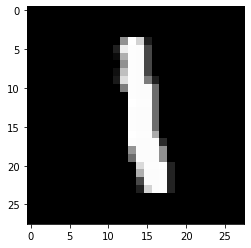``````import keras
import jovian``````
```Using TensorFlow backend. ```
``````import numpy as np

from keras.models import Sequential
from keras.layers import Dense, Dropout, Activation, Flatten, Add, BatchNormalization
from keras.layers import Convolution2D, MaxPooling2D, SeparableConv2D
from keras.utils import np_utils

from keras.datasets import mnist

from pprint import pprint``````
``(X_train, y_train), (X_test, y_test) = mnist.load_data()``
``````print (X_train.shape)
from matplotlib import pyplot as plt
%matplotlib inline
plt.imshow(X_train, cmap='gray')``````
```(60000, 28, 28) ```
``<matplotlib.image.AxesImage at 0x7f9d0900beb8>````````X_train = X_train.reshape(X_train.shape, 28, 28,1)
X_test = X_test.reshape(X_test.shape, 28, 28,1)``````